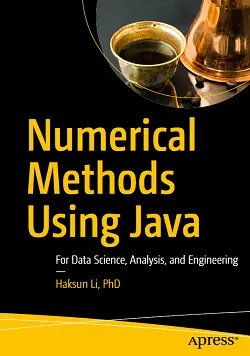# Numerical Methods Using Java### eBook Details:

• Paperback: 1200 pages
• Publisher: WOW! eBook (January 16, 2022)
• Language: English
• ISBN-10: 1484267966
• ISBN-13: 978-1484267967

### eBook Description:

Numerical Methods Using Java: For Data Science, Analysis, and Engineering

Implement numerical algorithms in Java using NM Dev, an object-oriented and high-performance programming library for mathematics. You’ll see how it can help you easily create a solution for your complex engineering problem by quickly putting together classes.

What You Will Learn

• Program in Java using a high-performance numerical library
• Learn the mathematics for a wide range of numerical computing algorithms
• Convert ideas and equations into code
• Put together algorithms and classes to build your own engineering solution
• Build solvers for industrial optimization problems
• Do data analysis using basic and advanced statistics

Numerical Methods Using Java covers a wide range of topics, including chapters on linear algebra, root finding, curve fitting, differentiation and integration, solving differential equations, random numbers and simulation, a whole suite of unconstrained and constrained optimization algorithms, statistics, regression and time series analysis. The mathematical concepts behind the algorithms are clearly explained, with plenty of code examples and illustrations to help even beginners get started.

[ Exclusive Offer! Order Intelligent EMS V Face Massager Now. Get Lowest Price & 60 Day Return Policy. Huge Discounts Available! Bravo Goods Special Offer Expires Soon. ]